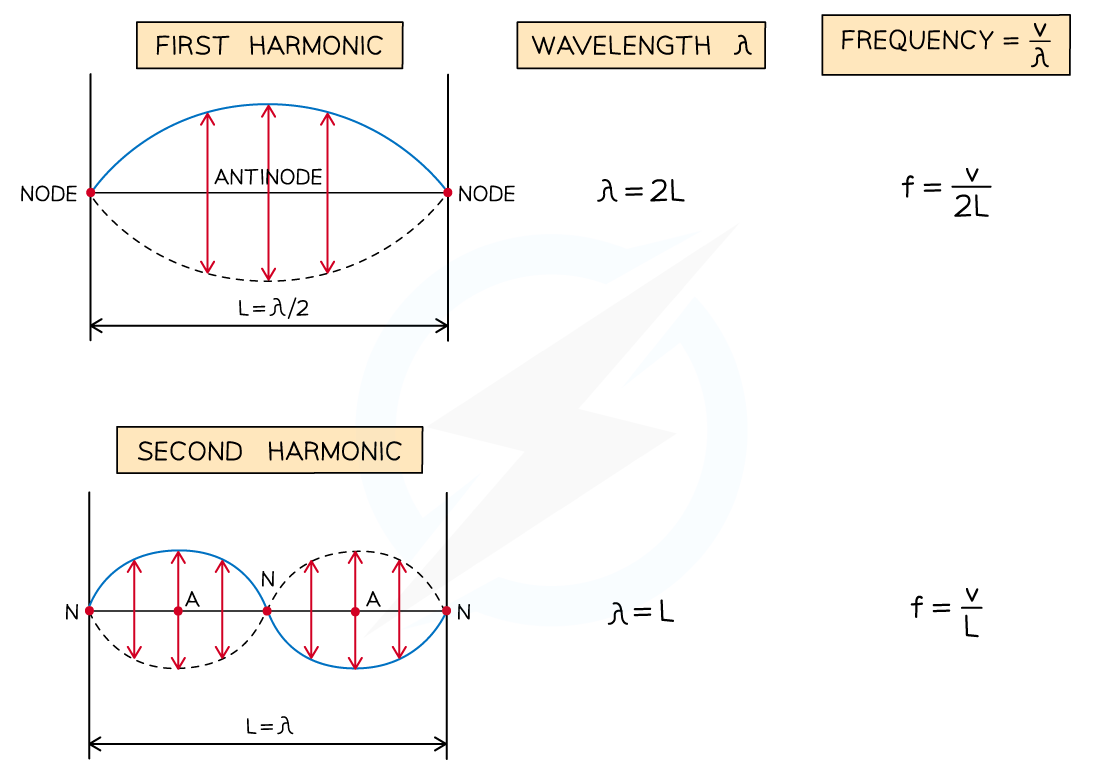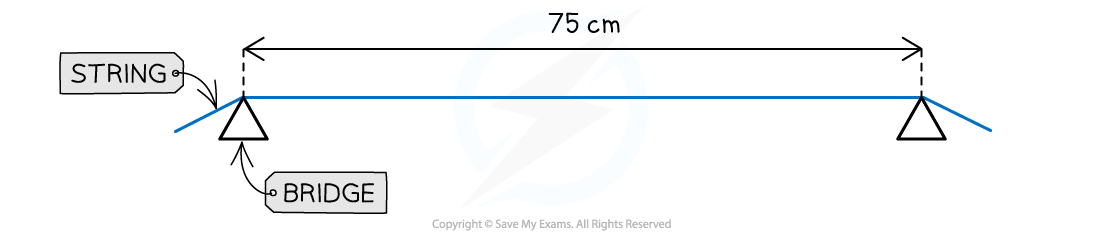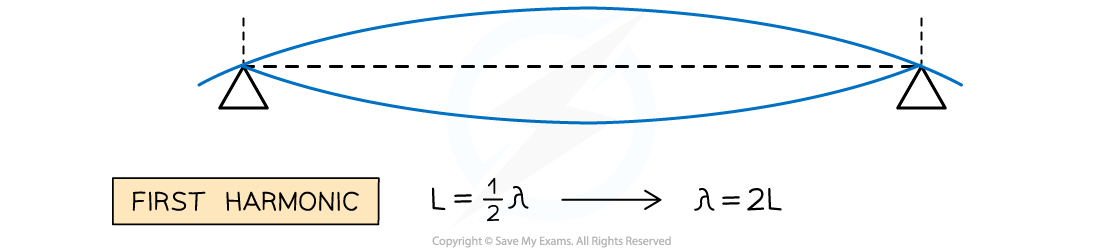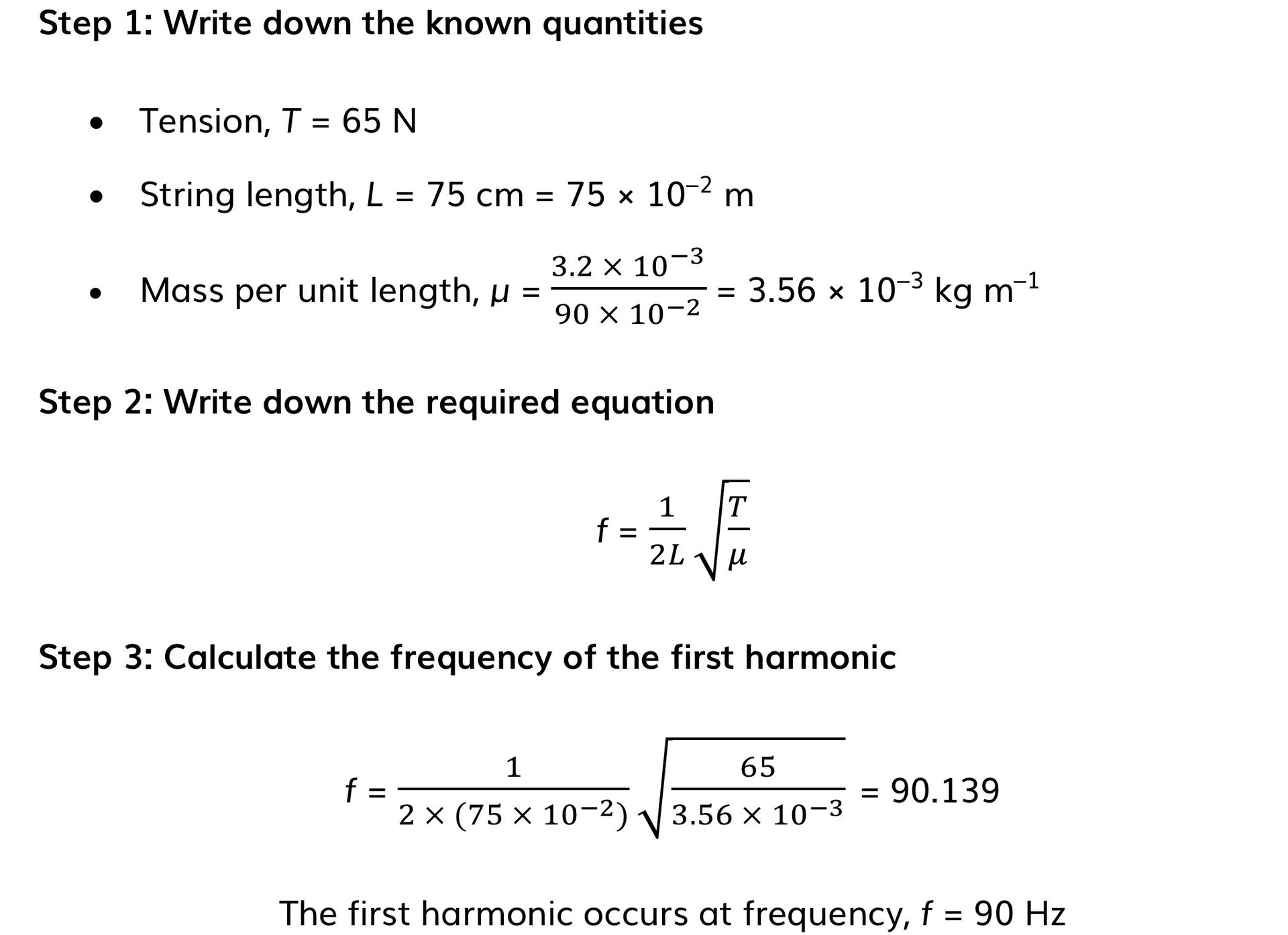# AQA A Level Physics复习笔记3.2.3 Harmonics

### Harmonics

• Stationary waves can have different wave patterns, known as harmonics
• These depend on the frequency of the vibration and the situation in which they are created

• These harmonics can be observed on a string with two fixed ends
• As the frequency is increased, more harmonics begin to appear

#### Harmonics on a String

• When a stationary wave, such as a vibrating string, is fixed at both ends, the simplest wave pattern is a single loop made up of two nodes and an antinode
• This is called the first harmonic

• The particular frequencies (i.e. resonant frequencies) of stationary waves formed depend on the length of the string L and the wave speed v
• The frequencies can be calculated from the string length and wave equation
• For a string of length L, the wavelength of the lowest harmonic is 2L
• This is because there is only one loop of the stationary wave, which is a half wavelength

• Therefore, the frequency is equal to:• The second harmonic has three nodes and two antinodes
• The wavelength is L and the frequency is equal to:• The third harmonic has four nodes and three antinodes
• The wavelength is 2L / 3 and the frequency is equal to:• The nth harmonic has n antinodes and n + 1 nodes
• The wavelengths and frequencies of the first three harmonics can be summarised as follows:Diagram showing the first three modes of vibration of a stretched string with corresponding frequencies

#### Worked Example

A stationary wave made from a string vibrating in the third harmonic has a frequency of 150 Hz.Calculate the frequency of the fifth harmonic

Step 1: Calculate the frequency of the first harmonic

f3 = 3 f1

f1 = f3 ÷ 3 = 150 ÷ 3 = 50 Hz

Step 2: Calculate the frequency of the fifth harmonic

f5 = 5 f1

f5 = 5 × 50 = 250 Hz

#### Exam Tip

Make sure to match the correct wavelength with the harmonic asked for in the question:

• The first harmonic (or n = 1) is the lowest frequency with half or quarter of a wavelength
• The second harmonic (or n = 2) is a full wavelength

### Frequency of the First Harmonic

• The speed of a wave travelling along a string with two fixed ends is given by:• Where:
• T = tension in the string (N)
• μ = mass per unit length of the string (kg m–1)

• For the first harmonic of a stationary wave of length L, the wavelength, λ = 2L
• Therefore, according to the wave equation, the speed of the stationary wave is:

v = fλ = f × 2L

• Combining these two equations leads to the frequency of the first harmonic:• Where:
• f = frequency (Hz)
• L = the length of the string (m)

#### Worked Example

A guitar string of mass 3.2 g and length 90 cm is fixed onto a guitar. The string is tightened to a tension of 65 N between two bridges at a distance of 75 cm.Calculate the frequency of the first harmonic produced when the string is plucked.